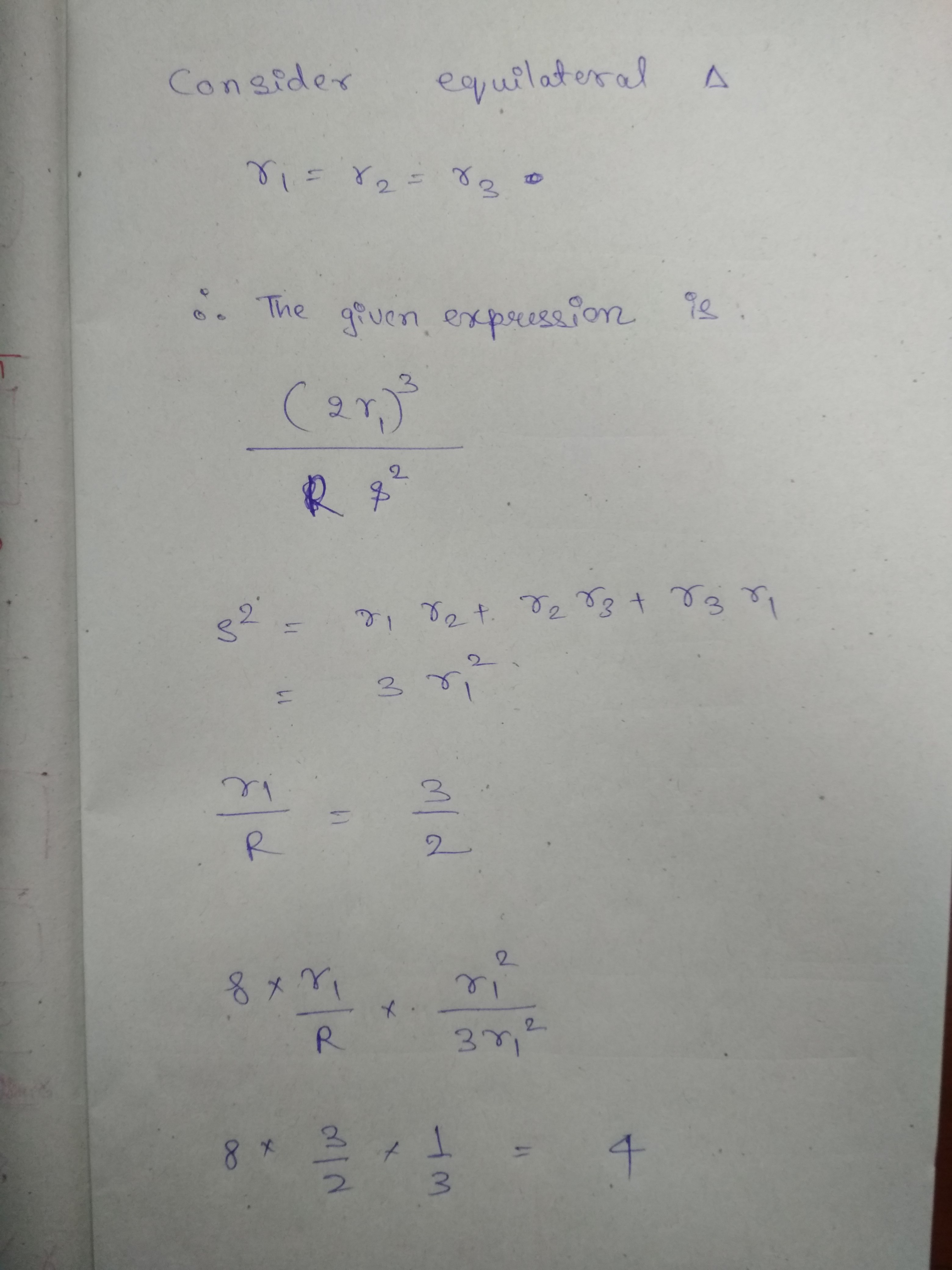Click to Chat

1800-1023-196

+91-120-4616500

CART 0

• 0

MY CART (5)

Use Coupon: CART20 and get 20% off on all online Study Material

ITEM
DETAILS
MRP
DISCOUNT
FINAL PRICE
Total Price: Rs.

There are no items in this cart.
Continue Shopping
`        My question is(r1+r2) (r2+r3) (r1+r3) divided by Rs^2 `
5 months ago

```							Dear student Yes it is divisible.I have attached the solution of this question as an image. Please check and let me know if any difficulty happen.```
5 months ago
```							dear aditi, aruns answer is totally crap and you shall get zero marks for such a solution. he has simply taken a special case of equilateral triangle. but we need to prove for general triangle.we know the standard formulas:r1= A/(s – a), r2= A/(s – b), r3= A/(s – c) where A is area of triangle and s is semiperimeter, 2s= a+b+cR= abc/4Aalso, by heron formulaA^2= s(s – a)(s – b)(s – c)substitute these values and simplify, we getA^3*abc/R(s(s – a)(s – b)(s – c))^2= 4A^4/(A^2)^2= 4A^4/A^4= 4kindly approve :))
```
5 months ago
Think You Can Provide A Better Answer ?

## Other Related Questions on Trigonometry

View all Questions »### Course Features

• 731 Video Lectures
• Revision Notes
• Previous Year Papers
• Mind Map
• Study Planner
• NCERT Solutions
• Discussion Forum
• Test paper with Video Solution### Course Features

• 31 Video Lectures
• Revision Notes
• Test paper with Video Solution
• Mind Map
• Study Planner
• NCERT Solutions
• Discussion Forum
• Previous Year Exam Questions